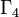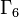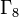# Linear representation theory of groups of order 2^n

This article gives specific information, namely, linear representation theory, about a family of groups, namely: groups of order 2^n.
View linear representation theory of group families | View other specific information about groups of order 2^n

This article describes the linear representation theory of groups of order 2^n, i.e., groups whose order is a power of$2$.$n$$2^n$ Number of groups of order$2^n$ Information on groups Information on linear representation theory
0 1 1 trivial group linear representation theory of trivial group
1 2 1 cyclic group:Z2 linear representation theory of cyclic group:Z2
2 4 2 groups of order 4 (see specifically cyclic group:Z4 and Klein four-group) linear representation theory of groups of order 4 (see specifically linear representation theory of cyclic group:Z4 and linear representation theory of Klein four-group)
3 8 5 groups of order 8 linear representation theory of groups of order 8
4 16 14 groups of order 16 linear representation theory of groups of order 16
5 32 51 groups of order 32 linear representation theory of groups of order 32
6 64 267 groups of order 64 linear representation theory of groups of order 64
7 128 2328 groups of order 128 linear representation theory of groups of order 128
8 256 56092 groups of order 256 linear representation theory of groups of order 256
9 512 10494213 groups of order 512 linear representation theory of groups of order 512
10 1024 49487365422 groups of order 1024 linear representation theory of groups of order 1024

## Degrees of irreducible representations

FACTS TO CHECK AGAINST FOR DEGREES OF IRREDUCIBLE REPRESENTATIONS OVER SPLITTING FIELD:
Divisibility facts: degree of irreducible representation divides group order | degree of irreducible representation divides index of abelian normal subgroup
Size bounds: order of inner automorphism group bounds square of degree of irreducible representation| degree of irreducible representation is bounded by index of abelian subgroup| maximum degree of irreducible representation of group is less than or equal to product of maximum degree of irreducible representation of subgroup and index of subgroup
Cumulative facts: sum of squares of degrees of irreducible representations equals order of group | number of irreducible representations equals number of conjugacy classes | number of one-dimensional representations equals order of abelianization
Number of degree 1 irreps Number of degree 2 irreps Number of degree 4 irreps Number of degree 8 irreps Total number of irreps Order of group (also, sum of squares of degrees) Number of groups with these degrees of irreps Description of the groups
1 0 0 0 1 1 1 trivial group only
2 0 0 0 2 2 1 cyclic group:Z2 only
4 0 0 0 4 4 2 Both the groups of order 4: cyclic group:Z4 and Klein four-group
8 0 0 0 8 8 3 The abelian groups of order 8: cyclic group:Z8, direct product of Z4 and Z2, elementary abelian group:E8
4 1 0 0 5 8 2 The non-abelian groups of order 8: dihedral group:D8 (see representation info) and quaternion group (see representation info)
16 0 0 0 16 16 5 The abelian groups of order 16: cyclic group:Z16, direct product of Z4 and Z4, direct product of Z4 and Z4, direct product of Z8 and Z2, direct product of Z4 and V4, elementary abelian group:E16
8 2 0 0 10 16 6 The groups of order 16, class exactly two, the Hall-Senior family$\Gamma_2$: SmallGroup(16,3), nontrivial semidirect product of Z4 and Z4, M16, direct product of D8 and Z2, direct product of Q8 and Z2, central product of D8 and Z4
4 3 0 0 7 16 3 The groups of order 16, class exactly three, the Hall-Senior family$\Gamma_3$: dihedral group:D16, semidihedral group:SD16, generalized quaternion group:Q16
32 0 0 0 32 32 7 All the abelian groups of order 32
16 4 0 0 20 32 15 The Hall-Senior family (up to isocliny)$\Gamma_2$
16 0 1 0 17 32 2 The extraspecial groups (Hall-Senior family$\Gamma_5$): inner holomorph of D8 and central product of D8 and Q8
8 6 0 0 14 32 19 The Hall-Senior families$\Gamma_3$ (ten groups, class three) and$\Gamma_4$ (nine groups, class two)
8 2 1 0 11 32 5 The Hall-Senior families$\Gamma_6$ and$\Gamma_7$
4 7 0 0 11 32 3 The maximal class groups (family$\Gamma_8$): dihedral group:D32, semidihedral group:SD32, generalized quaternion group:Q32

## Splitting field

### Important ways in which 2 differs from other primes

There are two very important differences between 2 and other primes:

• Odd-order and ambivalent implies trivial, so a nontrivial finite$p$-group for odd$p$ cannot be ambivalent, i.e., not all its characters are real-valued. In particular, this means that the representations cannot all be realized over the field of real numbers, and in particular, there are no nontrivial examples of rational-representation groups or rational groups for odd primes. However, for the prime$p = 2$, there are many examples of rational-representation groups (such as elementary abelian 2-groups and dihedral group:D8), examples of rational groups that are not rational-representation groups (such as quaternion group), and examples of other ambivalent groups (such as dihedral group:D16).
• Odd-order p-group implies every irreducible representation has Schur index one: This means that for an odd-order$p$-group, every irreducible representation can be realized over the field generated by its character values (Note: There do exist non-nilpotent odd-order groups with representations having Schur index values more than 1). This is not the case for 2-groups, and there exist irreducible representations of 2-groups with Schur index greater than 1. The smallest example is faithful irreducible representation of quaternion group, which has Schur index 2.
• The multiplicative group of$\mathbb{Z}/p^n\mathbb{Z}$ is cyclic for odd$p$, but is not cyclic for$p = 2, n \ge 3$. Thus, we can construct examples of finite 2-groups such that the Galois group of a minimal cyclotomic splitting field over$\mathbb{Q}$ is not cyclic, and this is not possible for odd$p$. Therefore, 2 is the only prime where it is possible to construct examples where the orbit structure on the irreducible representations differs from the orbit structure on the conjugacy classes under the action of a Galois group. (Examples, links need to be provided).